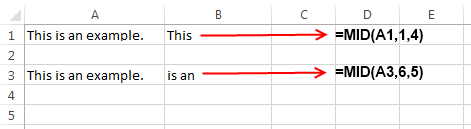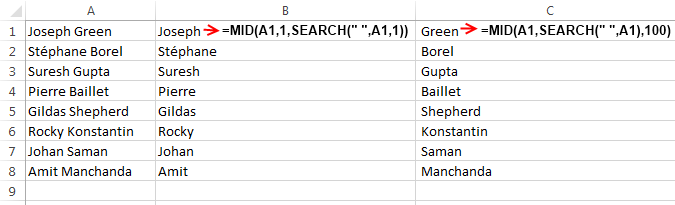# Excel MID Function – How to Use

Excel MID function is a replica of the substring function that you may have studied in programming languages. In simple words this formula is used to extract a small part of string from the input string or we can also define it as a function that returns a specified number of characters from the supplied string.

### How Excel defines MID Function:

Microsoft Excel defines MID function as a formula that “Returns the characters from the middle of a text string, given a starting position and length.”

This means that we have to supply MID function three things – 1. The source string 2. The starting position for extracting the substring and 3. The length of resultant string.

### Syntax of Excel MID Function:

The syntax of MID function is as under:

`=MID( Input_string, Start_position, Number_of_characters )`

‘Input_string’ signifies the string from which you want to extract a substring.

‘Start_position’ signifies the numerical position in the ‘Input_string’ from where you want to begin the extraction.

‘Number_of_characters’ indicates the number of characters that you wish to extract.

### Few Important things about MID Formula:

In MID Function the value of ‘Start_position’ should always be greater than ‘0’. If you enter its value less than or equal to ‘0’ then it results into a #VALUE! error.

In MID function the value of ‘Number_of_characters’ should always be greater than ‘0’. If its value is equal to ‘0’ then MID function will not extract any character and its output will be blank. If its value is less than ‘0’ then MID Function throws a #VALUE! error.

### Examples of MID Formula:In the above example I have used two MID functions:

1. In the first function I have used a formula `=MID(A1,1,4)` . So this formula starts extracting the substring from the input string present at ‘A1’. The extraction begins at position 1 and ends after 4 characters and hence the result a substring “This”.

2. In the second function the MID formula is used as: `=MID(A3,6,5)`. In this example the substring is extracted from the text present in ‘A3’ cell and the extraction begins from 6th position and ends after 5 characters and that’s why the resultant substring is “is an”.

### A better way to perform substrings using MID function:

As you have already seen that MID function works in a pretty hardcoded manner. By hardcoded, I mean in MID you have to specify the start position and the number characters yourself. What if, you have a list of people names along with their surnames separated by a space? How will you extract a person’s first name from such a list?

Now in such a case only using MID function is not going to help you as you cannot be sure about the number of characters in a person’s name or surname. In such situations Search Function can be quite helpful. It helps you to create better and sophisticated substring formulas.

If you are not aware of SEARCH formula then probably you should read this post.In the above example I have used MID function along with Search to extract a person’s First and Last name.

1. For Extracting First Name of a Person: I have used the formula `=MID(A1,1,SEARCH(" ",A1,1))` . In this example MID function searches the string at A1 position and starts the substring operation from 1st character of the input string. For the length of extracted string I have used Search Function which scans the string at A1 cell for the space (“ ”) character and returns its position as an integer value. And thus the resultant of both these functions fetches the person’s name.

2. For Extracting Last Name of a Person: In the second part to extract the last name of a person I have used the formula `=MID(A1,SEARCH(" ",A1),100)` . In this formula the MID function extracts from the cell no. A1. The start position is decided by the Search formula, here Search formula gives the position of space character (“ ”) and hence extraction starts from there and continues till the end of string.

The above function also includes a space (“ ”) character at the start of the Last name substring so a better option would be to use `=MID(A2,SEARCH(" ",A1)+1,100)`.About Ankit Kaul

Ankit is the founder of Excel Trick. He is tech Geek who loves to sit in front of his square headed girlfriend (his PC) all day long. :D. Ankit has a strong passion for learning Microsoft Excel. His only aim is to turn you guys into 'Excel Geeks'.

1.shivpalsays

I have a table as below”
no. pass
1005 y
2005 n
3005 y
4005 y
7248 y

I want the output like this:

Roll No. of Pass candidates are as below:
1005 3005 4005 7248

this output should come by using a formula, where the rown can be thousands and each roll no. contains 11 digit. and also columns are more than 50.

I can made it by using mid function but i had to repeat the command and space is not enough to repeat too much in a cell.

I am using the function
=mid(if(istext(‘sheet’!b1),’sheet1!a1′,””,)&if(istext(‘sheet1′!b2),” “&’sheet1!a2’,””)

can you suggest any looping.

•Ankit Kaulsays

Hi Shivpal, If you are looking for looping then the obvious approach is to go for VBA macros.
I have sent you an email containing a spreadsheet where I have used a small macro to achieve the desired result.
Hope it helps. 🙂

2.Vsays

Hi,

I m going to extract text in the middle of a text with specific characters as limit to how many characters it will extract.

Example:
was/star/watercharges
was/petron/watercharges

I want to extract star only or petron only, how can i extract star and petron using mid? since it always require the number of characters to extract, how about the variation of number of characters i will require in the formula??

•Ankit Kaulsays

Hi V,
You can try using the formula `=MID(MID(A1,FIND("/",A1)+1,100),1,FIND("/",MID(A1,FIND("/",A1)+1,100))-1)`
This formula will extract the middle part of a text string (with “/” as delimiter).
Please let me know if you need any other info from my end 🙂

3.vadisays

Hi!
I have a list of number with a space ,I have to elimine the space between the numbers, how can i use the mid funtion to do that went you have to change a 300 numbers like this? the list is like this:
00 24 35 26 35 52
00 35 65 26 85 45
00 45 65 26 85 78

I need change like this:
002435263552
003565268545
004565268578
thanks

•Ankit Kaulsays

This can be easily done by using SUBSTITUTE function.
You can simply write the formula as:
`=SUBSTITUTE(A1," ","")` [where ‘A1’ contains the text from which spaces are to be removed]
In case you need more details on this function then you can see this article.
Hope it helps 🙂

•vadisays

Thanks a lot for your help
it works!!!!!!!

•Ankit Kaulsays

4.Jaysonsays

Hi,

I have a problem with number starting with zero. I also need to capture the zero.
For example. 0987 the result only display 987.
How can i get the zero?
What function appropriate to use?
thanks.

•Ankit Kaulsays

Hi Jason, this is because Excel is treating them as numbers.
Just change the format of those cells to text and then Excel won’t remove the starting 0.
Hope it helps 🙂

5.johnsays

How can you nest two mid functions? For example, I have two columns

731481xx-Genie 07-Bottle = i want the data to extract only = 73148107

any help is appreciated

•Ankit Kaulsays

In this case the formula would be: `=MID(A1,1,6)&MID(B1,1,2)`
Provided A1 contains “731481xx-Genie” and B1 contains “07-Bottle”.

Also, I have assumed that the number of digits, you want to extract will always remain same
i.e. 6 digits from A1 cell and 2 digits from B1 cell.
Hope it helps 🙂